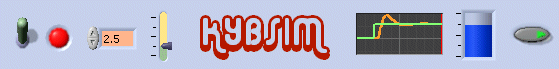# FREQUENCY RESPONSE ANALYSIS OF CONTROL SYSTEM

## Description of the simulated system

The control system of a process given by a transfer function model is simulated. You can adjust the setpoint and the disturbance. The frequency response of the control system is shown in a Bode diagram.

### The controlled process

The process model which is controlled in this simulator can be chosen you. By default the transfer function from control variable, u, to measurement signal, y, is a second order transfer function with time-delay, with a disturbance, v, acting on the process at the same place dynamically as the control variable. More specifically, the default process model is

y(s) = Hps(s)u(s) + Hps(s)v(s)

where

Hps(s) = [1/(s^2 + 2s + 1)]e-0.5s)

### The nominal operating point

The nominal operating point is characterized as follows:

• The process measurement ym is 50 (in a proper unit)
• The disturbance is 10.
• The nominal control signal, u0, which keeps the process in or close to the operating point, is 40.

### Controller function

The PID controller is on serial form, i.e. its transfer function is

Hc(s) = Kp [(1+Tis)(1+Tds)]/[Tis(Tfs+1)]

## Aim

The aim of this simulator is to give practice in frequency response based analysis of feedback control systems.

## Motivation

The frequency response is a good tool for expressing the dynamic properties of a control system, regarding setpoint tracking and disturbance compensation, and stability.

In the tasks below it is assumed that the process is in the nominal operating point, which is defined above.

1. Controller tuning:

Find proper PID settings using e.g. Ziegler-Nichols' closed-loop method (the sustained oscillations method or the frequency response method).

Unless otherwise stated, it is assumed that you use a PID controller with these settings in the tasks below.

2. Static setpoint tracking:
1. Characterize qualitatively the setpoint tracking property of the control system as read off from both the tracking function and the sensitivity function.
2. Confirm the results in subtask a by running a simulation (apply steps in the setpoint and the disturbance).

3. Dynamic setpoint tracking:
1. What is the -3dB bandwidth?
2. Run a proper simulation which hopefully confirms that the bandwidth is as found in subtask a above.
3. Run simulations which demonstrate that the tracking is good "below" the bandwidth, and poor above the bandwidth.
4. Assume the setpoint tracking response time Tr from the bandwidth. (The response time is gthe 63% rise time of the step response, analog to the time constant of a first order system.) Tr can be estimated as

Tr = 1.5/wb.

Compare with the response time as found from a simulation. Are the values similar?

5. What is the bandwidth and the response time for a properly tuned PI controller? Compare with the PID controller.

4. Static disturbance compensation:
1. Explain from the shape of |S| (sensitivity function) in a Bode plot why the control system gives perfect static disturbance compensation.
2. Confirm the result in subtask a by running a proper simulation.

5. Dynamic disturbance compensation:
1. Let the setpoint be constant (50). Run the following two simulations with a sinusoidal disturbance of frequency wv less than the bandwidth (e.g. 0.3rad/s): In the first simulation the control loop shall be open (i.e. the controller is in manual mode). In the second simulation the controller the control loop is closed (i.e. the controller is in automatic modus). Calculate from the simulations the ratio of the respective amplitudes in the response in ym. Is this ratio in accordance with the |S(jw)| plot?
2. As in subtask a above, but the disturbance frequency shall now be larger than the bandwidth (e.g. 5 rad/s).

6. Stability:
1. What are the stability margins (gain margin GM and phase margin PM) with the PID controller (tuned in task 1)? Do they have proper values?
2.
1. Increase the controller gain Kp until the control system becomes marginally stable as seen from the time response. (Keep this value Kp in the following subtasks.)
2. What does the tracking function |T| and the sensivity function |S| look like (at marginal stability)?
3. Read off the amplitude crossover frequency and the phase crossover frequency. Do they have equal values?
4. Calculate the period of the time response from the wc value. Confirm the result by a simulation.
5.
3. Reset the PID controller to proper settings (as found in task 1).
1. Increase the controller gain Kp until the control system gets poor stability. How are the |T| and |S| curves changed? (Then reset Kp.)
2. Increase the process time delay until the control system gets poor stability. How are the |T| and |S| curves changed?

[KYBSIM] [TechTeach]

Updated October 4, 2004. Developed by Finn Haugen. E-mail: finn@techteach.no.